Скачать презентацию Case Studies SYSTEMC Slide -1 — TRANSACTION-LEVEL

• Количество слайдов: 25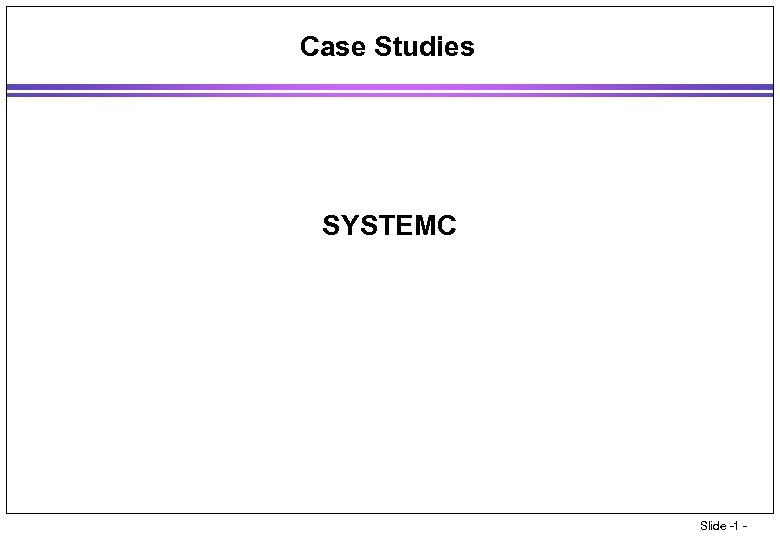Case Studies SYSTEMC Slide -1 -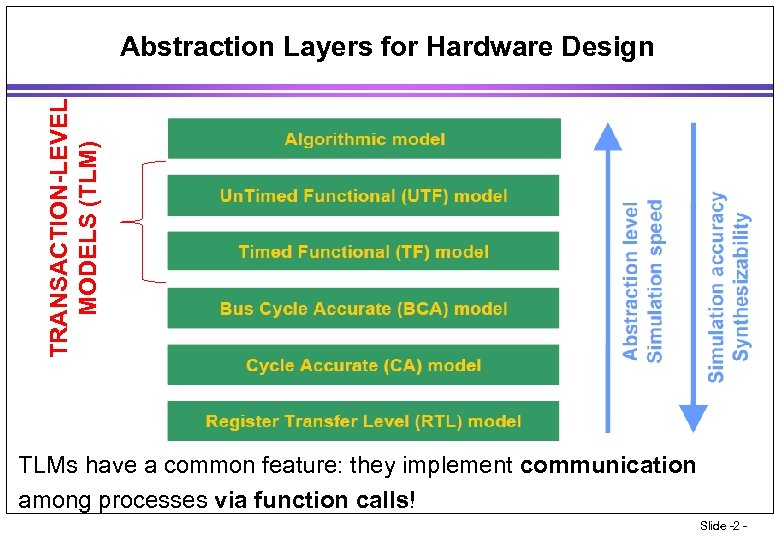TRANSACTION-LEVEL MODELS (TLM) Abstraction Layers for Hardware Design • TLMs have a common feature: they implement communication • among processes via function calls! Slide -2 -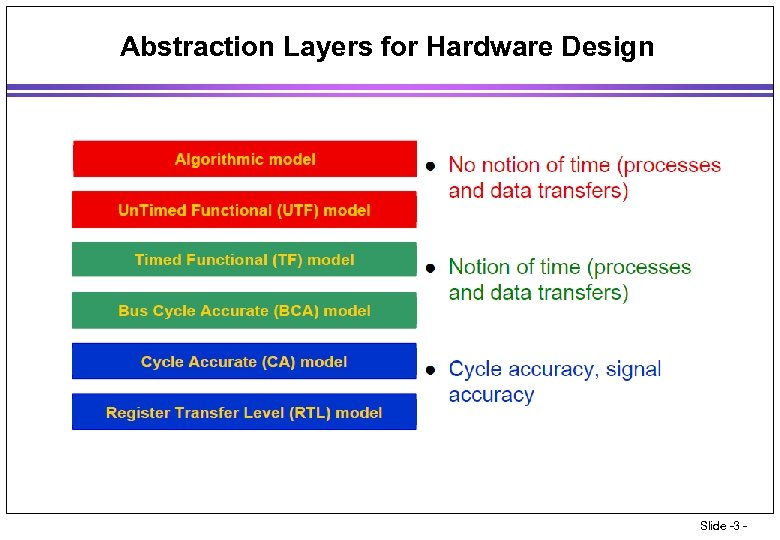Abstraction Layers for Hardware Design Slide -3 -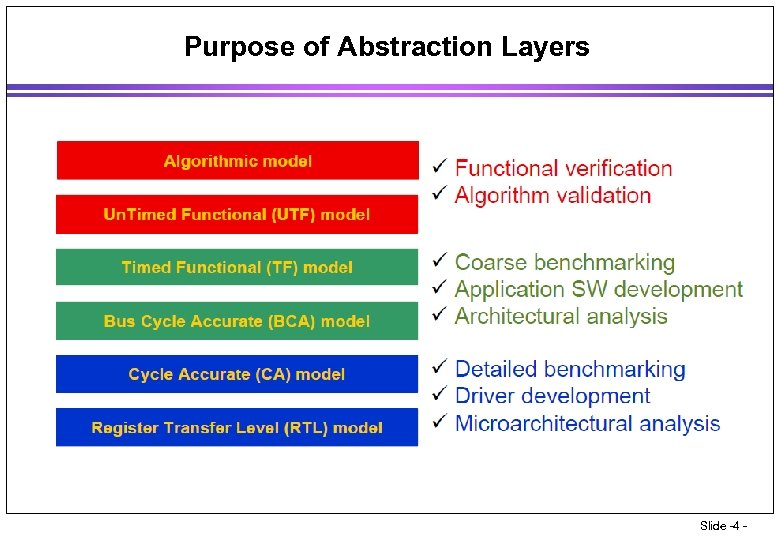Purpose of Abstraction Layers Slide -4 -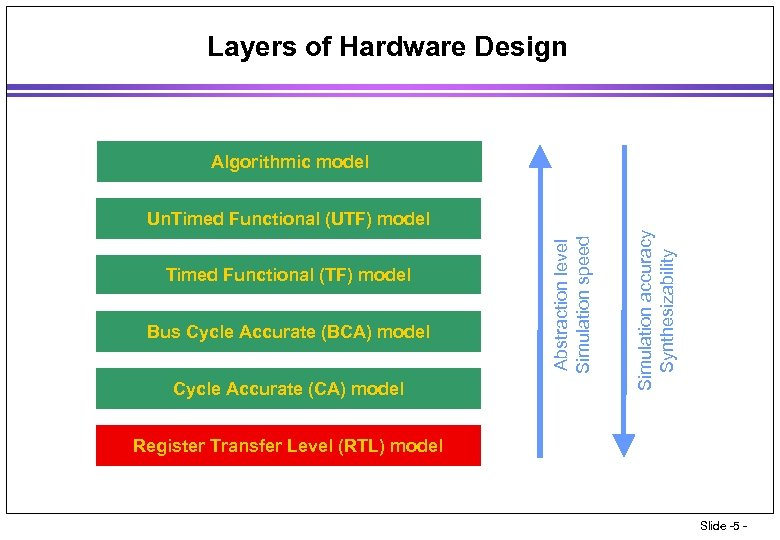Layers of Hardware Design Algorithmic model Bus Cycle Accurate (BCA) model Cycle Accurate (CA) model Simulation accuracy Synthesizability Timed Functional (TF) model Abstraction level Simulation speed Un. Timed Functional (UTF) model Register Transfer Level (RTL) model Slide -5 -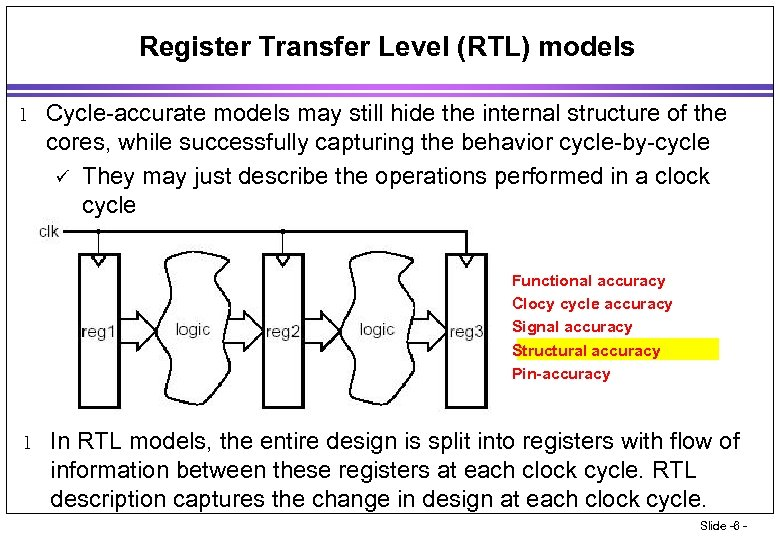Register Transfer Level (RTL) models l Cycle-accurate models may still hide the internal structure of the cores, while successfully capturing the behavior cycle-by-cycle ü They may just describe the operations performed in a clock cycle • Functional accuracy • Clocy cycle accuracy • Signal accuracy • Structural accuracy • Pin-accuracy l In RTL models, the entire design is split into registers with flow of information between these registers at each clock cycle. RTL description captures the change in design at each clock cycle. Slide -6 -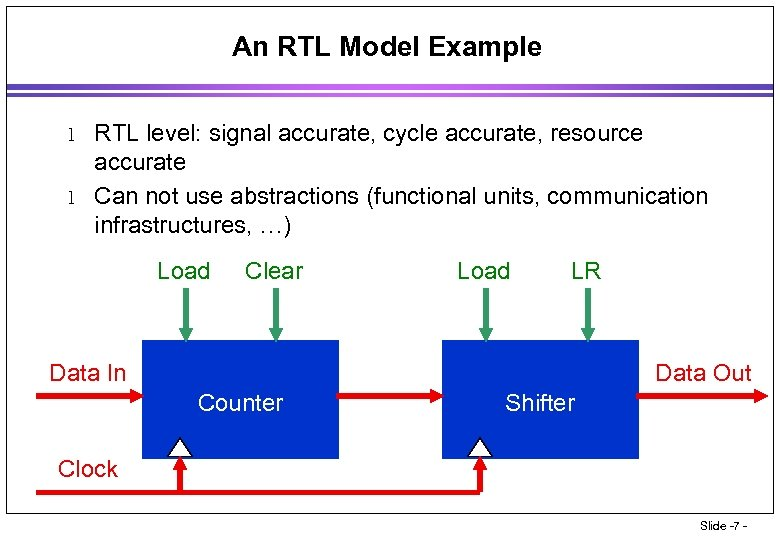An RTL Model Example l l RTL level: signal accurate, cycle accurate, resource accurate Can not use abstractions (functional units, communication infrastructures, …) Load Clear Load LR Data In Data Out Counter Shifter Clock Slide -7 -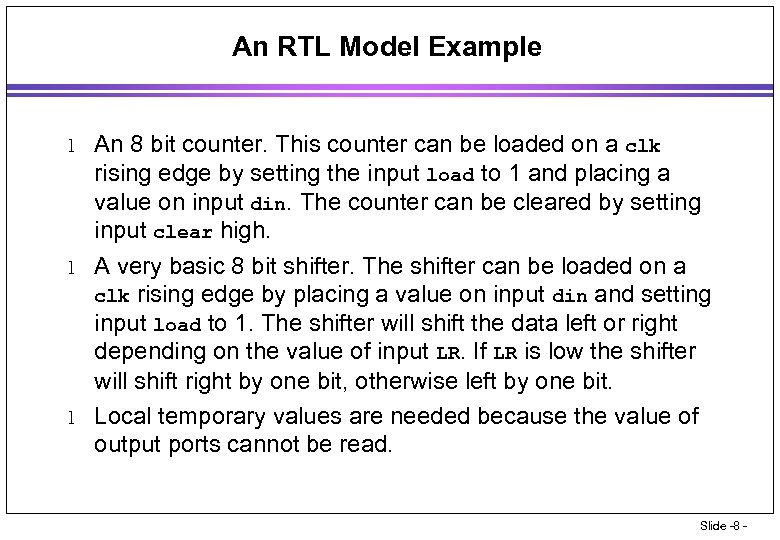An RTL Model Example l l l An 8 bit counter. This counter can be loaded on a clk rising edge by setting the input load to 1 and placing a value on input din. The counter can be cleared by setting input clear high. A very basic 8 bit shifter. The shifter can be loaded on a clk rising edge by placing a value on input din and setting input load to 1. The shifter will shift the data left or right depending on the value of input LR. If LR is low the shifter will shift right by one bit, otherwise left by one bit. Local temporary values are needed because the value of output ports cannot be read. Slide -8 -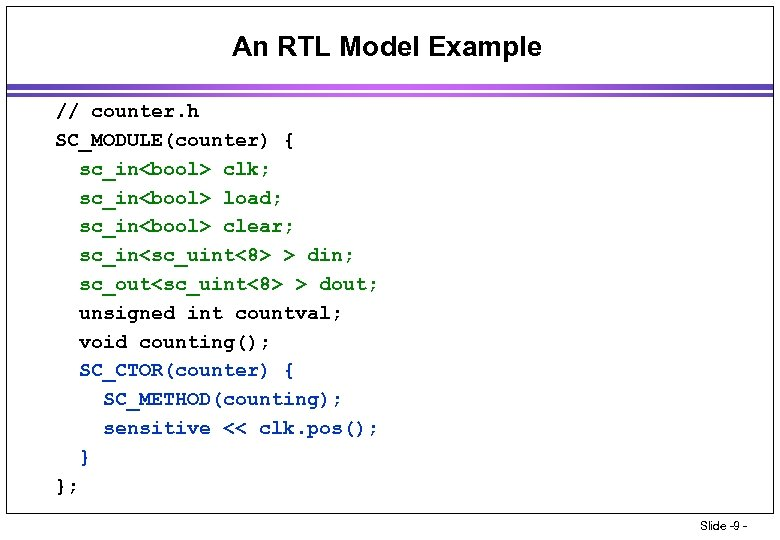An RTL Model Example // counter. h SC_MODULE(counter) { sc_in clk; sc_in load; sc_in clear; sc_in > din; sc_out > dout; unsigned int countval; void counting(); SC_CTOR(counter) { SC_METHOD(counting); sensitive << clk. pos(); } }; Slide -9 -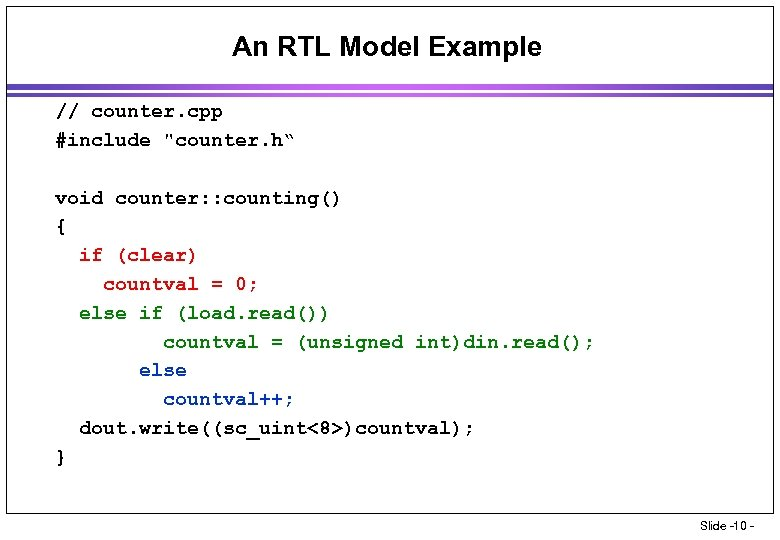An RTL Model Example // counter. cpp #include "counter. h“ void counter: : counting() { if (clear) countval = 0; else if (load. read()) countval = (unsigned int)din. read(); else countval++; dout. write((sc_uint<8>)countval); } Slide -10 -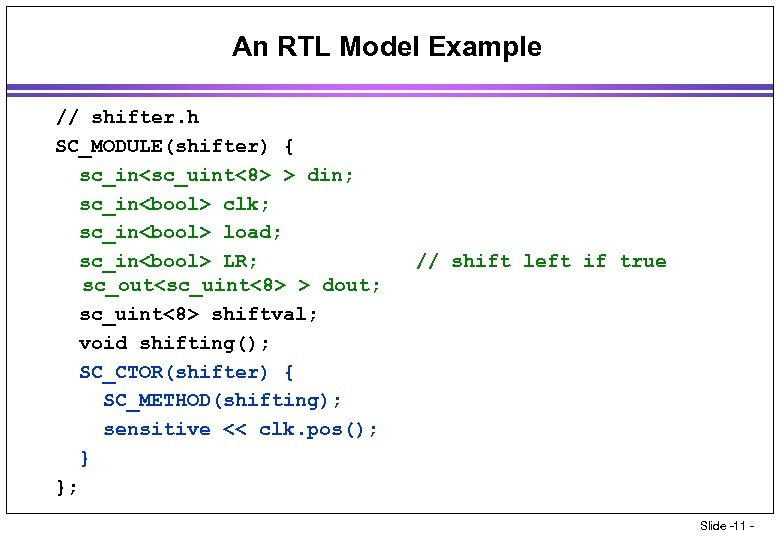An RTL Model Example // shifter. h SC_MODULE(shifter) { sc_in > din; sc_in clk; sc_in load; sc_in LR; sc_out > dout; sc_uint<8> shiftval; void shifting(); SC_CTOR(shifter) { SC_METHOD(shifting); sensitive << clk. pos(); } }; // shift left if true Slide -11 -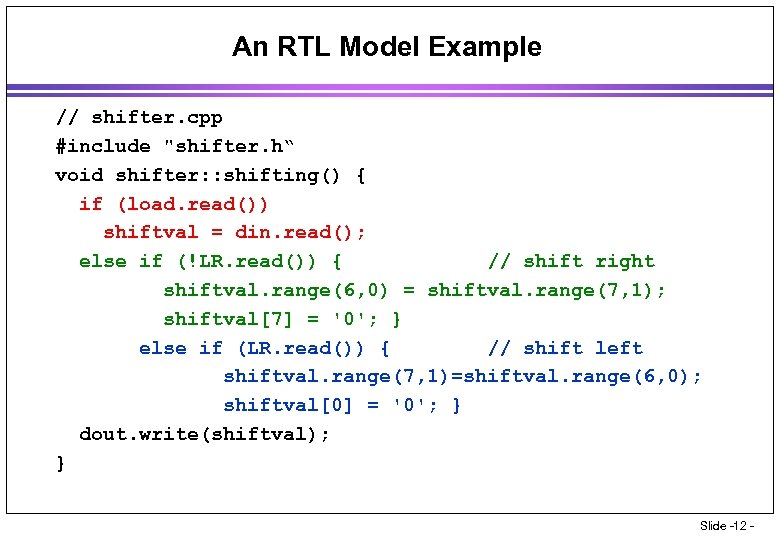An RTL Model Example // shifter. cpp #include "shifter. h“ void shifter: : shifting() { if (load. read()) shiftval = din. read(); else if (!LR. read()) { // shift right shiftval. range(6, 0) = shiftval. range(7, 1); shiftval = '0'; } else if (LR. read()) { // shift left shiftval. range(7, 1)=shiftval. range(6, 0); shiftval = '0'; } dout. write(shiftval); } Slide -12 -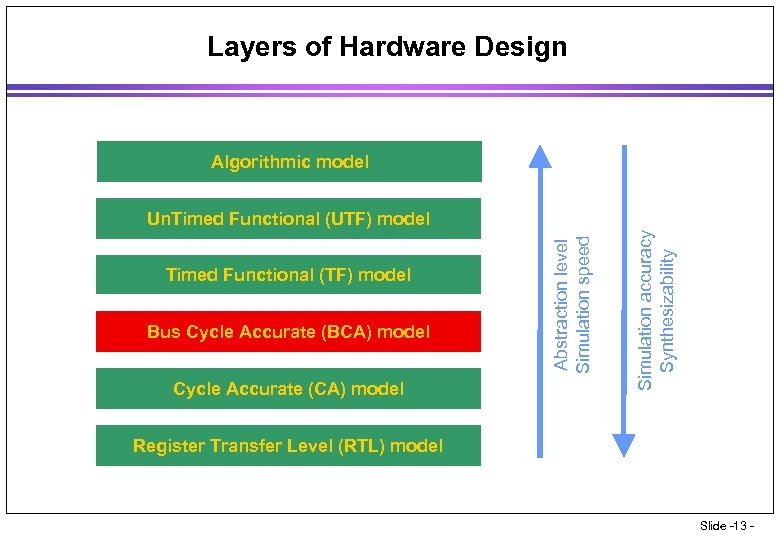Layers of Hardware Design Algorithmic model Bus Cycle Accurate (BCA) model Cycle Accurate (CA) model Simulation accuracy Synthesizability Timed Functional (TF) model Abstraction level Simulation speed Un. Timed Functional (UTF) model Register Transfer Level (RTL) model Slide -13 -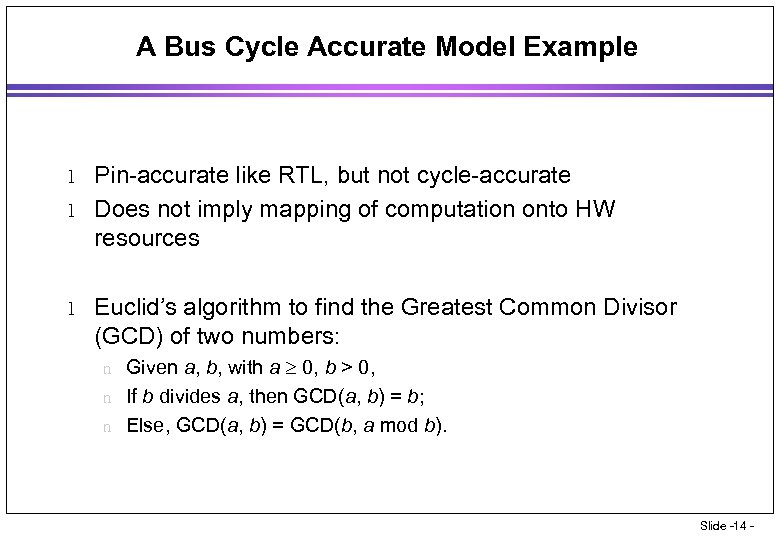A Bus Cycle Accurate Model Example l l l Pin-accurate like RTL, but not cycle-accurate Does not imply mapping of computation onto HW resources Euclid’s algorithm to find the Greatest Common Divisor (GCD) of two numbers: Given a, b, with a 0, b > 0, n If b divides a, then GCD(a, b) = b; n Else, GCD(a, b) = GCD(b, a mod b). n Slide -14 -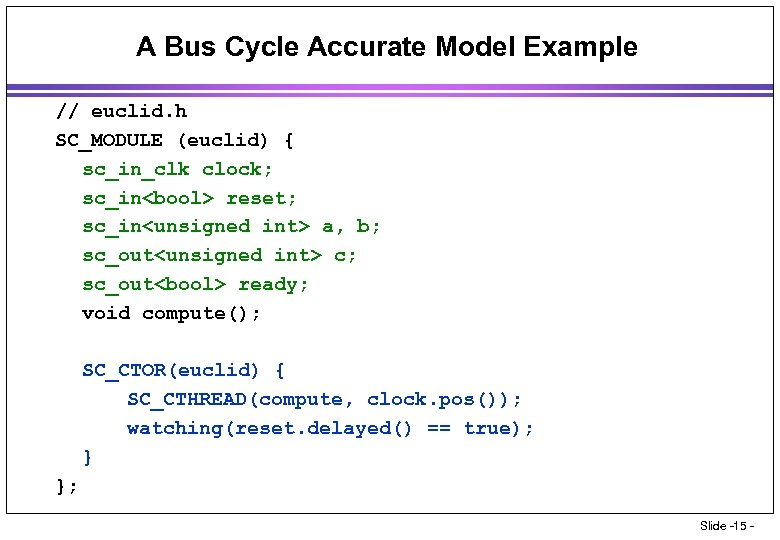A Bus Cycle Accurate Model Example // euclid. h SC_MODULE (euclid) { sc_in_clk clock; sc_in reset; sc_in a, b; sc_out c; sc_out ready; void compute(); SC_CTOR(euclid) { SC_CTHREAD(compute, clock. pos()); watching(reset. delayed() == true); } }; Slide -15 -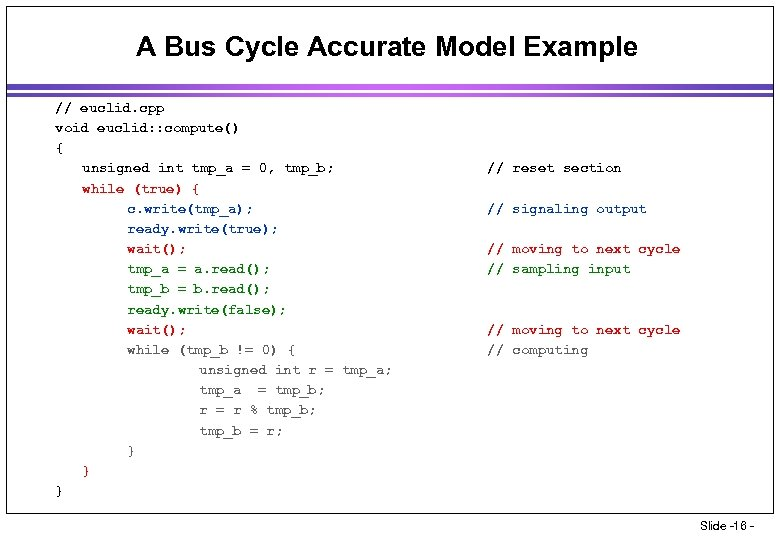A Bus Cycle Accurate Model Example // euclid. cpp void euclid: : compute() { unsigned int tmp_a = 0, tmp_b; while (true) { c. write(tmp_a); ready. write(true); wait(); tmp_a = a. read(); tmp_b = b. read(); ready. write(false); wait(); while (tmp_b != 0) { unsigned int r = tmp_a; tmp_a = tmp_b; r = r % tmp_b; tmp_b = r; } } } // reset section // signaling output // moving to next cycle // sampling input // moving to next cycle // computing Slide -16 -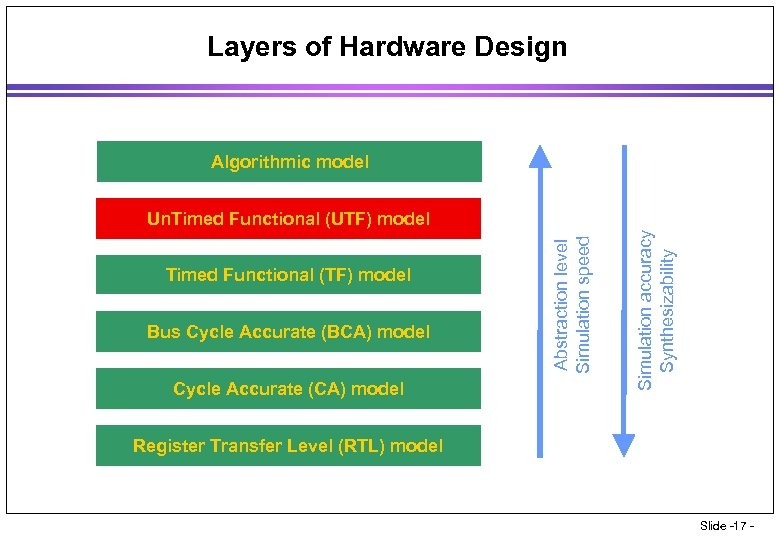Layers of Hardware Design Algorithmic model Bus Cycle Accurate (BCA) model Cycle Accurate (CA) model Simulation accuracy Synthesizability Timed Functional (TF) model Abstraction level Simulation speed Un. Timed Functional (UTF) model Register Transfer Level (RTL) model Slide -17 -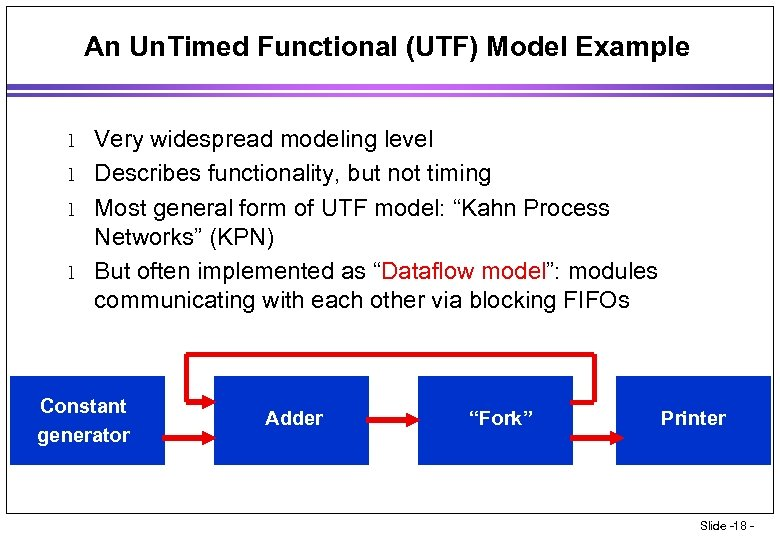An Un. Timed Functional (UTF) Model Example l l Very widespread modeling level Describes functionality, but not timing Most general form of UTF model: “Kahn Process Networks” (KPN) But often implemented as “Dataflow model”: modules communicating with each other via blocking FIFOs Constant generator Adder “Fork” Printer Slide -18 -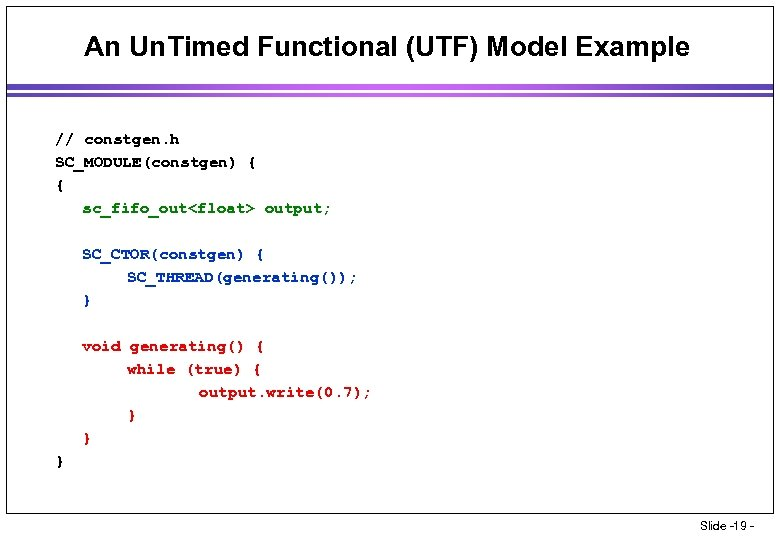An Un. Timed Functional (UTF) Model Example // constgen. h SC_MODULE(constgen) { { sc_fifo_out output; SC_CTOR(constgen) { SC_THREAD(generating()); } void generating() { while (true) { output. write(0. 7); } } } Slide -19 -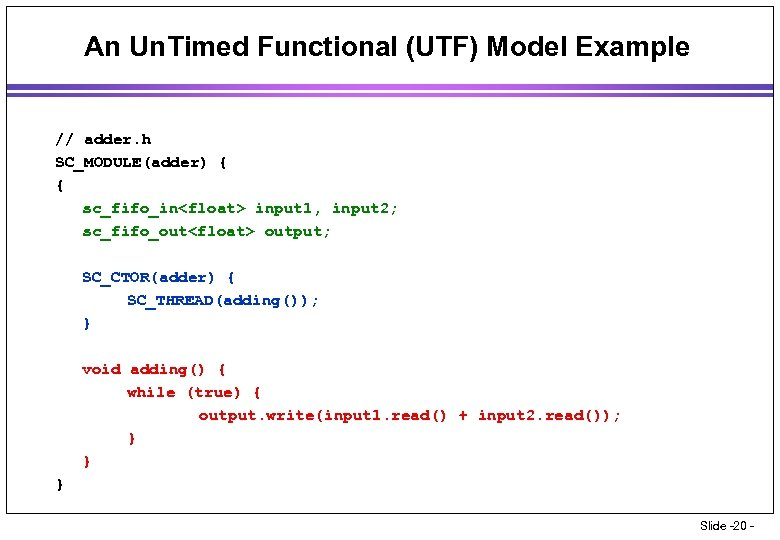An Un. Timed Functional (UTF) Model Example // adder. h SC_MODULE(adder) { { sc_fifo_in input 1, input 2; sc_fifo_out output; SC_CTOR(adder) { SC_THREAD(adding()); } void adding() { while (true) { output. write(input 1. read() + input 2. read()); } } } Slide -20 -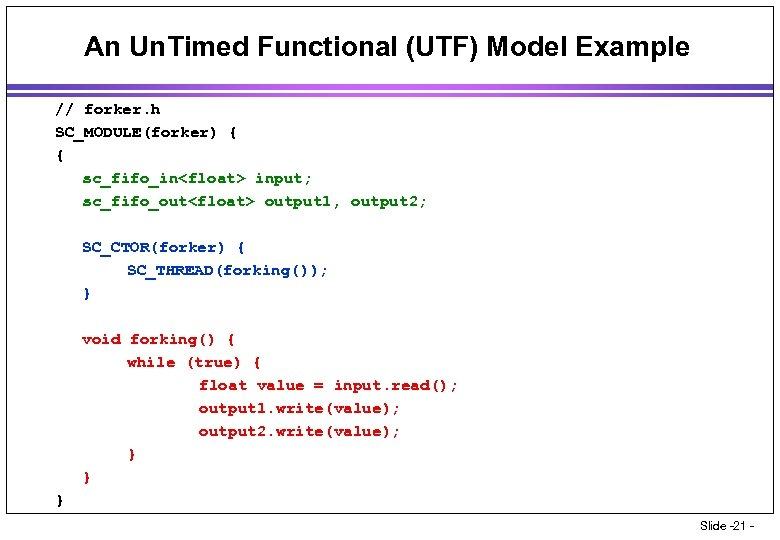An Un. Timed Functional (UTF) Model Example // forker. h SC_MODULE(forker) { { sc_fifo_in input; sc_fifo_out output 1, output 2; SC_CTOR(forker) { SC_THREAD(forking()); } void forking() { while (true) { float value = input. read(); output 1. write(value); output 2. write(value); } } } Slide -21 -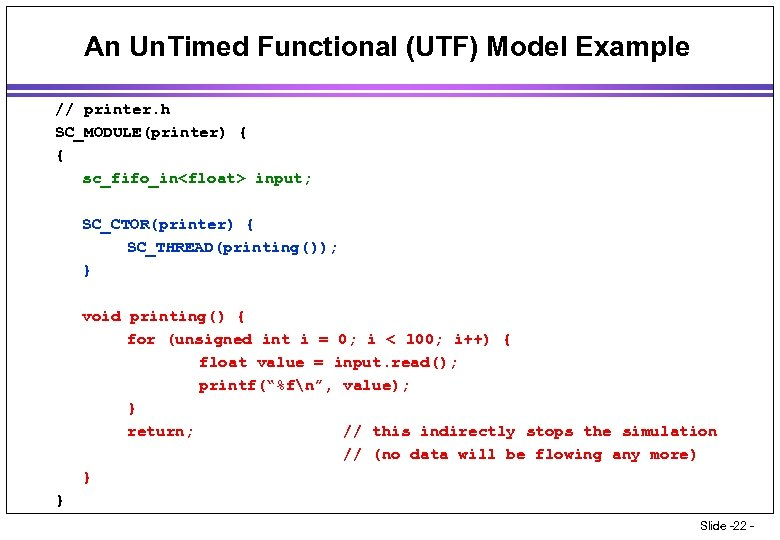An Un. Timed Functional (UTF) Model Example // printer. h SC_MODULE(printer) { { sc_fifo_in input; SC_CTOR(printer) { SC_THREAD(printing()); } void printing() { for (unsigned int i = 0; i < 100; i++) { float value = input. read(); printf(“%fn”, value); } return; // this indirectly stops the simulation // (no data will be flowing any more) } } Slide -22 -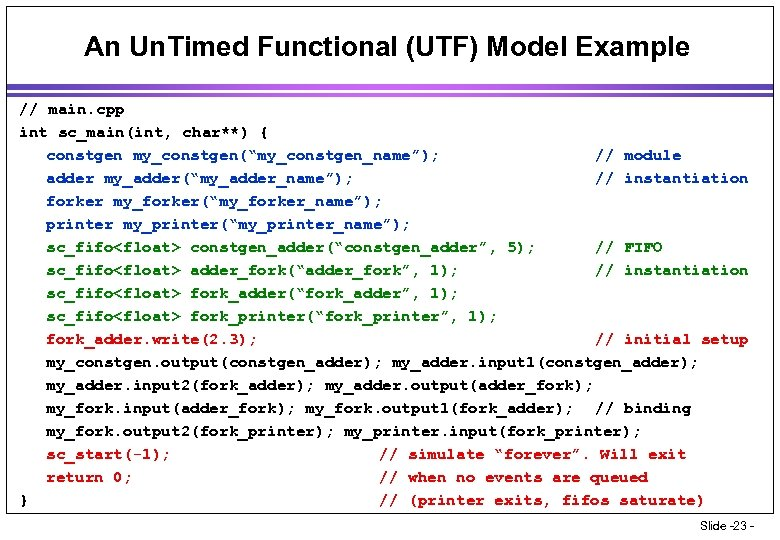An Un. Timed Functional (UTF) Model Example // main. cpp int sc_main(int, char**) { constgen my_constgen(“my_constgen_name”); // module adder my_adder(“my_adder_name”); // instantiation forker my_forker(“my_forker_name”); printer my_printer(“my_printer_name”); sc_fifo constgen_adder(“constgen_adder”, 5); // FIFO sc_fifo adder_fork(“adder_fork”, 1); // instantiation sc_fifo fork_adder(“fork_adder”, 1); sc_fifo fork_printer(“fork_printer”, 1); fork_adder. write(2. 3); // initial setup my_constgen. output(constgen_adder); my_adder. input 1(constgen_adder); my_adder. input 2(fork_adder); my_adder. output(adder_fork); my_fork. input(adder_fork); my_fork. output 1(fork_adder); // binding my_fork. output 2(fork_printer); my_printer. input(fork_printer); sc_start(-1); // simulate “forever”. Will exit return 0; // when no events are queued } // (printer exits, fifos saturate) Slide -23 -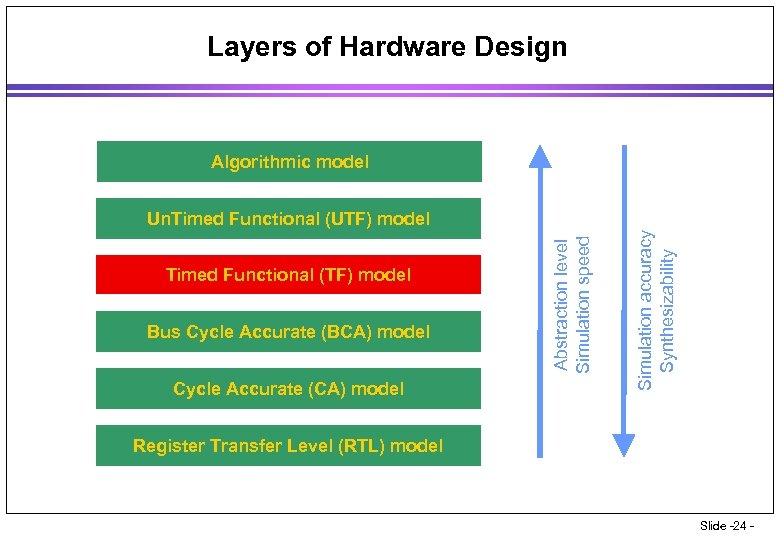Layers of Hardware Design Algorithmic model Bus Cycle Accurate (BCA) model Cycle Accurate (CA) model Simulation accuracy Synthesizability Timed Functional (TF) model Abstraction level Simulation speed Un. Timed Functional (UTF) model Register Transfer Level (RTL) model Slide -24 -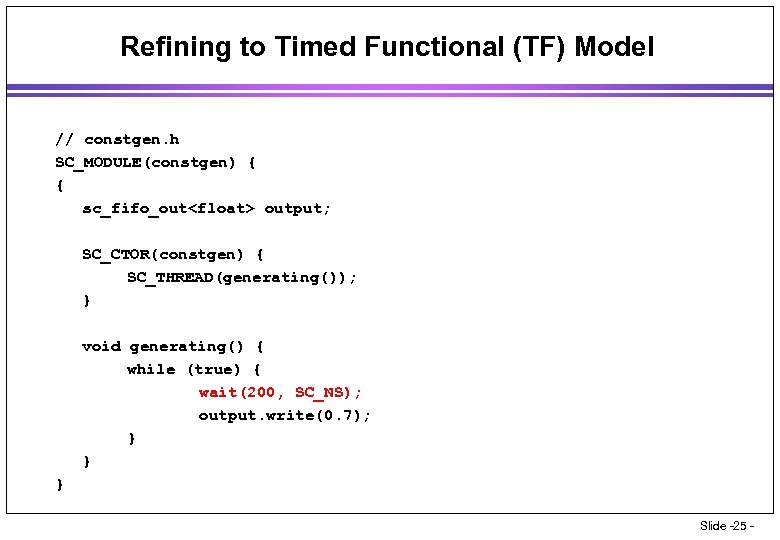Refining to Timed Functional (TF) Model // constgen. h SC_MODULE(constgen) { { sc_fifo_out output; SC_CTOR(constgen) { SC_THREAD(generating()); } void generating() { while (true) { wait(200, SC_NS); output. write(0. 7); } } } Slide -25 -